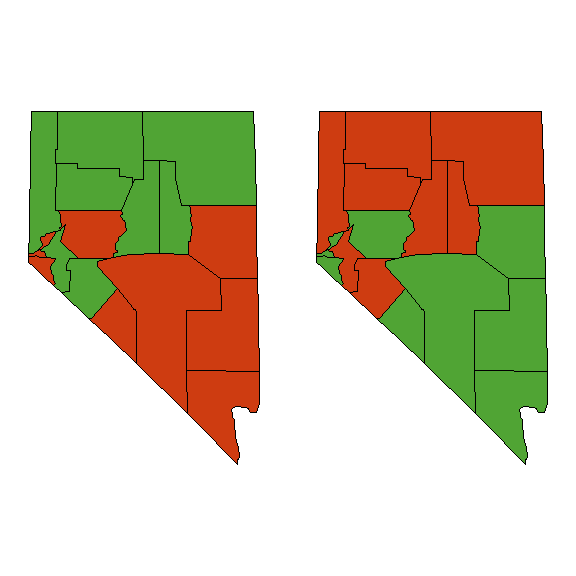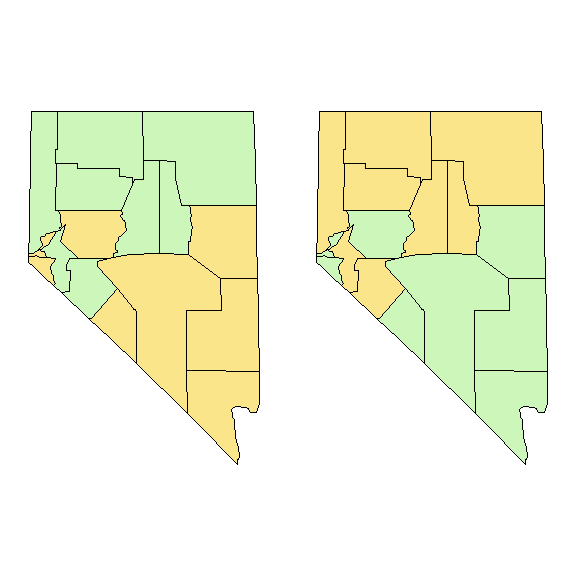A Color-Caused Optical Illusion

2019-12-04

Cleveland and McGill (1983) conducted experiments with different partitionings of colored-areas that were nearly the same size. In the example below, the two colored regions are less tha 1% different in area.

Using a map (similar to the one below left) with regions coded by two saturated colors, 49% of subjects said the red area was bigger, 22% said the green was bigger, and 31% said the areas were the same.

library(maps)
#library(mapproj)
#nv <- map('county', 'nevada', fill=TRUE, col=1:12, projection="mollweide")
nv.names <- c(reds,grns)

# Draw the map and color it
nv.names <- map('county', 'nevada', namesonly=TRUE, plot=FALSE)

# green/red
op <- par(mar=c(0,1,0,1), mfrow=c(1,2))
col=ifelse(nv.names %in% reds,
rgb(206, 60, 17, max=255), # red
rgb(80,164,52, max=255)), # green
projection="mollweide")
# red/green
col=ifelse(nv.names %in% reds,
rgb(80,164,52, max=255),
rgb(206, 60, 17, max=255)),
projection="mollweide")par(op)
# Area of red region = 55581
sum(area.map(nv, regions=reds))
##  55581.16
# Area of green region = 55687
sum(area.map(nv, regions=grns))
##  55686.61

Muted colors

When low-saturated, muted colors were used, the observers demonstrated no systematic biases.

op <- par(mar=c(0,1,0,1), mfrow=c(1,2))
# green/tan
col=ifelse(nv.names %in% reds,
rgb(250, 229, 139, max=255),
rgb(204, 246, 186, max=255)),
projection="mollweide")
# tan/green